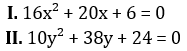Dear Aspirants,

Quantitative Aptitude Quiz For SBI Clerk

Numerical Ability or Quantitative Aptitude Section has given heebie-jeebies to the aspirants when they appear for a banking examination. As the level of every other section is only getting complex and convoluted, there is no doubt that this section, too, makes your blood run cold. The questions asked in this section are calculative and very time-consuming. But once dealt with proper strategy, speed, and accuracy, this section can get you the maximum marks in the examination. Following is the Quantitative Aptitude quiz to help you practice with the best of latest pattern questions.

Directions (1-5): In each of the following questions two equations are given. You have to solve the equations and Give answer –

Q1.if x < y
if x ≤ y
relationship between x and y cannot be determined
if x ≥ y
if x > y

Q2.if x < y
if x ≤ y
relationship between x and y cannot be determined
if x ≥ y
if x > y

Q3.if x < y
if x ≤ y
relationship between x and y cannot be determined
if x ≥ y
if x > y

Q4.if x < y
if x ≤ y
relationship between x and y cannot be determined
if x ≥ y
if x > y

Q5.if x < y
if x ≤ y
relationship between x and y cannot be determined
if x ≥ y
if x > y

Directions (6-10): What approximate value will come in place of question mark (?) in the given questions: (You are not expected to calculate the exact value.)

Q6. 619.992 – 134.99 ÷ 14.998 – (9.01)² =?

720
530
650
690
490
Solution:
? = 619.992 – 134.99 ÷ 14.998 – (9.01)² ≈ 620 – 135 ÷ 15 – (9)² ≈ 530

Q7. 449.97 ÷ 15.02 + 208.08 ÷ 8.01 – 16.01 =?
120
60
100
80
40
Solution:
? = 449.97 ÷ 15.2 + 208.08 ÷ 8.01 – 16.01 ≈ 450 ÷ 15 + 208 ÷ 8 – 16
= 30 + 26 – 16 = 30 + 10 = 40

Q8.4
5
2
3
1

Q9. ? % of (140.06 × 7.99 – 679.92) = 330.01
75
90
80
50
60

Q10. 40% of 859 + 87.89 ÷ 7.99 =?
398
286
412
215
355

Directions (11-15): The following bar graph shows the total number of management students from 5 different universities who attended a seminar organized by IIM Ahamadabad in year 2017. Study the graph and answer the questions that follows :Q11. What is the average number of boys who participated in the seminar?

6,600
6,400
6,250
6,845
6,125

Q12. The number of boys from AKTU who participated in seminar is what percent of number of girls from Indraprasth university who participated in seminar?
120%
160%
150%
130%
100%

Q13. What is the difference between average number of boys from all five universities together and average number of girls from all five universities together who attended the seminar?
2,750
2,800
2,850
2,500
2,150

Q14. What is the ratio of number of girls from LPU and AKTU together to the number of girls from DU and BBD together who attended the seminar?
7 : 5
5 : 7
9 : 11
11 : 9
3 : 7

Q15. The number of boys who attended the seminar from DU and AKTU together is approximately what percent more or less than the number of girls from AKTU and Indraprasth together who attended the seminar?
55% less
55% more
50% more
52% less
50% less

You May also like to Read: